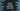# Python Tutorial : Part 2 : Variables### Python Variable :

Variable is a reserved memory location to store values. You can assume it as a bucket that contains a value. Names of the different variable should be different and unique. The rules for writing variable name is the same as we have seen for identifiers.

### Assigning a value :

In python, equal ( = ) sign is used to assign values to a variable. You don’t need to specify what type of variable this is ( e.g integer, float etc.), it will happen automatically. Also no need to declare explicitly to reserve a memory location for a variable, you can directly assign a value.

E.g :

``````
count = 10
print(count)
``````

It will print 10

### Reassigning a variable :

Can you reassign a variable? i.e. currently count is an integer and it has a value 10. Can we convert count to a String variable? Yes, we can. This is the beauty of python :

``````count = 10

count = "This is a String"
print(count)
``````

The result will be “This is a String”.

### Multiple Assignment :

Multiple Assignment means assigning different values to different variables in a single line. In python, we can achieve this like below :

``````a, b, c = 5, 2, 1
a, b, c = 5, 2.0, ”different values"
``````

So, you can assign different values or the same value to several variables simultaneously.

### Delete a variable :

You can also delete a variable using “del” command. Let’s take a look at the following example :

``````count = 100
del count

print(count)
``````

If you will try to run the above script, the following error msg will be shown :

``NameError: name 'count' is not defined.``

### Local and Global Variables :

Global variables are accessible from anywhere in your program. But a Local variable can be accessed only inside a function or method where it is declared. Let’s take a look at the following program :

``````myString = "Global String"

def innerFunction():
myString = "Local String"
print(myString)

innerFunction()
print(myString)
``````

It will first print “Local String”. myString defined inside innerFunction() is a local variable. So on calling innerFunction(), it will take the value of the local variable.

``````myString = "Global String"

def innerFunction():
#myString = "Local String"
print(myString)

innerFunction()
print(myString)
``````

What about now? It will print “Global String” twice. As we have commented out the local variable declaration part, it will take the value from the global String.

``````myString = "Global String"

def innerFunction():
global myString
myString = "Local String"
print(myString)

innerFunction()
print(myString)
``````

This time? It will print Local String two times. That means we can access Global variables using global keyword and change it inside a function.

``````myString = "Global String"

def innerFunction():
print myString
myString = "Local String"
print(myString)

innerFunction()
print(myString)
``````

### The output will be :

``UnboundLocalError: local variable 'myString' referenced before assignment.``

Why? Because we have created one variable myString inside innerFunction. So, python will assume that the first print statement is trying to print the local variable, which is not defined yet and it will throw the above error message.

### Concatenation :

``````count = 100
statement = "Print a number"

print(statement,count)
print(statement+count)
``````

### Result :

``The second print statement will throw an error : TypeError: cannot concatenate 'str' and 'int' objects``

So, first, we need to change count to a String variable before concatenating it with another String variable. Let’s check :

``````count = 100
statement = "Print a number"

print(statement,count)
print(statement+str(count))
``````

### Output :

``````Print a number 100
Print a number100
``````

Only difference is that the first statement has a space between “number” and “100”. The first print statement is actually not a concatenation. We are just passing different arguments to the print function and print joins them with a separator keyword . ( Default is space ).